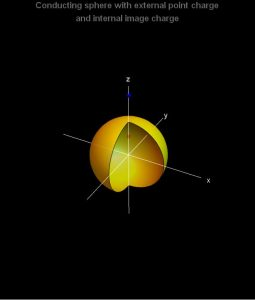# Electrodynamics

I add four notebooks dealing with electrostatic topics and calculations as treated in Jackson Classical Electrodynamics.17-42 Jackson Electrostatics - I 09-16-17

This is the first of four notebooks dealing with electrostatic topics and calculations as treated in Jackson Classical Electrodynamics. This notebook focuses on the classic problem of determining the potential associated with a point charge outside a grounded spherical conductor, a calculation appearing in most textbooks. In this notebook, the problem is solved using the method of images. In subsequent notebooks, this same problem will be treated in other ways.

or

17-43 Jackson Electrostatics - 2 09-16-17

This is the second of four notebooks dealing with electrostatic topics and calculations as treated in Jackson Classical Electrodynamics. This notebook focuses on an electrostatic problem with axi-symmetry that is solved using an appropriate Green’s function. The treatment starts with a summary of Jackson’s solution of the Poisson equation for the Green’s function. Then the Green’s function is identified with the potential for a conducting sphere in the presence of an external charge – obtained using the method of images in Jackson Electrostatics – 1 09-16-17. The Green’s function formalism is applied to the problem of a conducting sphere with hemispheres held at different potentials.

or

17-44 Jackson Electrostatics - 3 09-17-17

This is the third of four notebooks dealing with electrostatic topics and calculations as treated in Jackson Classical Electrodynamics. This notebook focuses on the expansion of 1/|r – rhat| in terms of a power series of eigenfunctions, and its application to the specific problem of determining the potential associated with a ring of charge.

or

17-45 Jackson Electrostatics - 4 09-17-17

This is the last of four notebooks dealing with electrostatic topics and calculations as treated in Jackson Classical Electrodynamics. This notebook focuses on the solution of Poisson’s equation for Green’s function in spherical coordinates. A general form for the Green’s function satisfying Dirichlet boundary conditions is obtained in the region between two concentric spherical conductors. Some special cases are obtained by calculating limits of the general case.  This is the last of four notebooks dealing with electrostatic topics and calculations as treated in Jackson Classical Electrodynamics. This notebook focuses on the solution of Poisson’s equation for Green’s function in spherical coordinates. A general form for the Green’s function satisfying Dirichlet boundary conditions is obtained in the region between two concentric spherical conductors. Some special cases are obtained by calculating limits of the general case.

Applications of these results are made to three problems

• Conducting sphere with external point charge
• Conducting sphere with external ring of charge
• Conducting sphere with internal line charge